Disclosure: This post may contain affiliate links, meaning when you click the links and make a purchase, we receive a commission.

# How to calculate Average, Median, & Mode in Excel

The average of a set of numbers is referred to as the arithmetic mean and it is calculated by adding all the numbers in the data set and then dividing by the count of the actual numbers in the data set.

The median describes the value which is at the center of the data set, basically. Half the numbers are greater than the median and the other half are less than the median. The mode describes the value which occurs most often in a specific dataset. Later versions of Excel have two mode functions namely MODE.SNGL and MODE.MULT. MODE.SNGL returns a single value and this value is the most frequent value in the dataset.

MODE.MULT returns a vertical array of the most frequently occurring values in a set. Sometimes data sets have more than one mode, so the MODE.MULT function accommodates this situation.

There are some advantages to each indicator. With the average, the advantage is that all the numbers in the data set are incorporated, in the final computation. The disadvantage of the average is that very large or very small values can distort the average value. These values are referred to as outliers and they have the most significant impact on the average value. The median is not affected by outliers as much as the average is, which is an advantage. However, for very large data sets such as those obtained from a census, the median can take a long time to compute.

The advantage of the mode is that it is not affected by outliers as dramatically as the average either. However, at times a data set may not contain a mode.

So, let’s get started with a simple example to illustrate the different measures of central tendency.

Read More: How to Use Compound Interest Formula in Excel

## Introduction

The Hip-Hop word count database was the brainchild of Harvard University fellow, Tahir Hemphill. He has been conceptualizing and developing this database since 2006. Currently, the database contains more than 50,000 rap songs from 1979 to the present day. A specially designed search engine that is incorporated into the database, allows users to search for highly nuanced information.

For champagne, for example, using the database one can pinpoint the exact year Moët superseded Dom Pérignon in popularity, in rap songs, which was in 1993.

One can also glean information such as whether Nike or Adidas dominates in mentions in rap songs. The influence of rap on pop culture and as an indicator of societal movements is increasingly being investigated in a data analysis context.

In our example, a hypothetical music producer wanting to create a hit rap song based on best practices gleaned from popular rap music of the past is analyzing a sheet containing data. A hypothetical list of the top selling, number one rap songs that went platinum within a designated three-year period are used as the source data, which is shown below: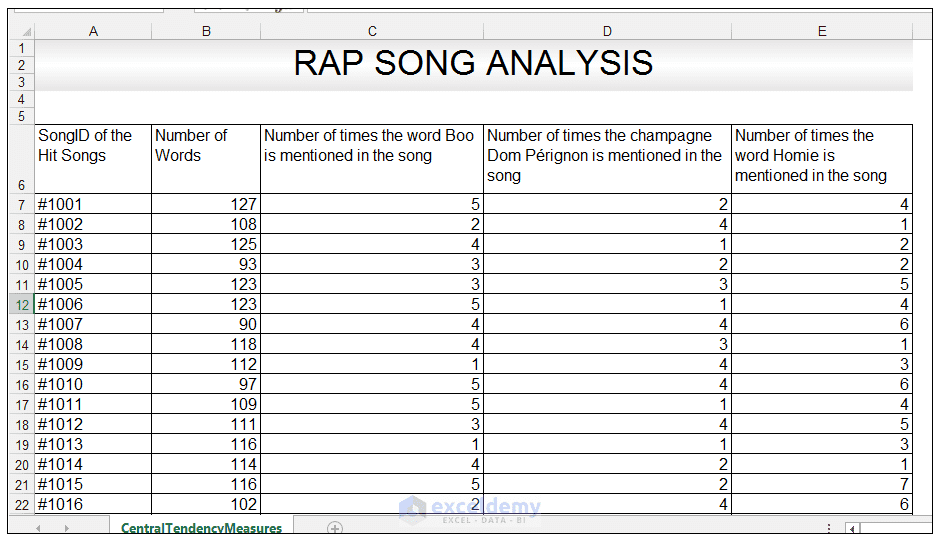There are five columns in the data set, namely the SongID of the Hit Songs column, The number of Words (in the song) column, the number of times the word Boo is mentioned in the song column, the Number of times the champagne Dom Pérignon is mentioned in the song column and the Number of times the word Homie is mentioned in the song. Boo is a colloquial way of referring to one’s girlfriend/boyfriend and homie is a colloquial way of referring to a friend or an acquaintance from one’s neighborhood. Champagne brands are often associated with a higher income/style of living and can be used as a device (other devices include mention of certain cars that the rapper is now driving) of noting the effects of passing a turning point in a rapper’s life and of the subsequent associated  lifestyle change past the turning point.

1) The first descriptive statistical measure, we are going to evaluate, is the average number of words of songs in this data set. So in Cell G8, we enter the formula and press CTRL-ENTER:

=AVERAGE(B7: B50)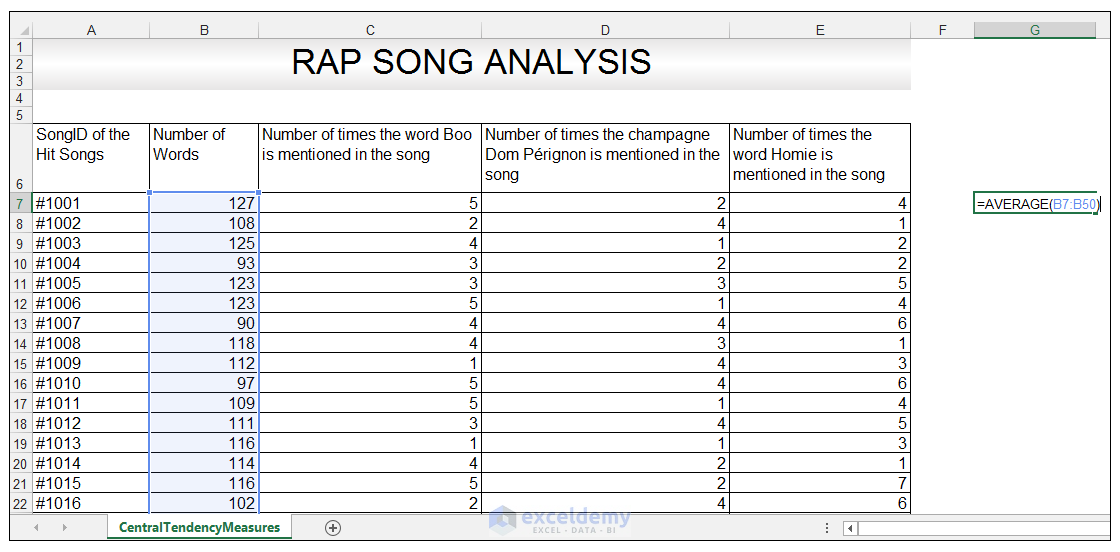The average function delivers the value 113.1, which means the average number of words was 113 words, for the hit songs under review.

Read More: Running Average: How to Calculate Using Excel’s Average(…) Function

2) We are now going to look at evaluating the median. In an even set of numbers, the median is determined by taking the average of the two middle numbers in the data set. We are going to use the Number of times the word Boo is mentioned in the song column. We want to see what the median, i.e the halfway point in terms of number for this column is. There are 44 number entries for this column.

So in Cell G9, we enter the following formula, and press CTRL-ENTER:

=MEDIAN(C7: C50)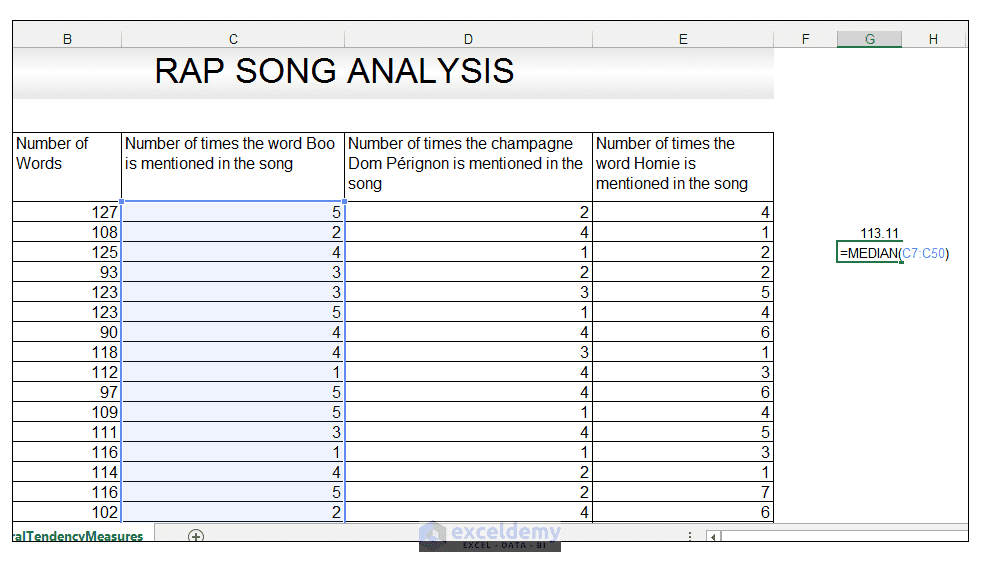The median function delivers the value of 3, which means that half of our values are below 3 and the other half are above 3. This means that half the songs on our hit list, mentioned the word “Boo” less than three times, and the other half mentioned the word Boo more than three times.

3) We are now going to look at evaluating the mode. Later versions of Excel allow one to calculate a single mode denoted by the MODE.SNGL function, and a MODE.MULT function. The MODE.SNGL function allows one to calculate the single most repetitive value occurring in the data set whereas the MODE.MULT returns a vertical array of the most frequently occurring values in a set of data. We are going to calculate the single most frequently occurring value in the Number of times the champagne Dom Pérignon is mentioned in the song.

So in Cell G10, we enter the following formula, and press CTRL-ENTER:

=MODE.SNGL(D7: D50)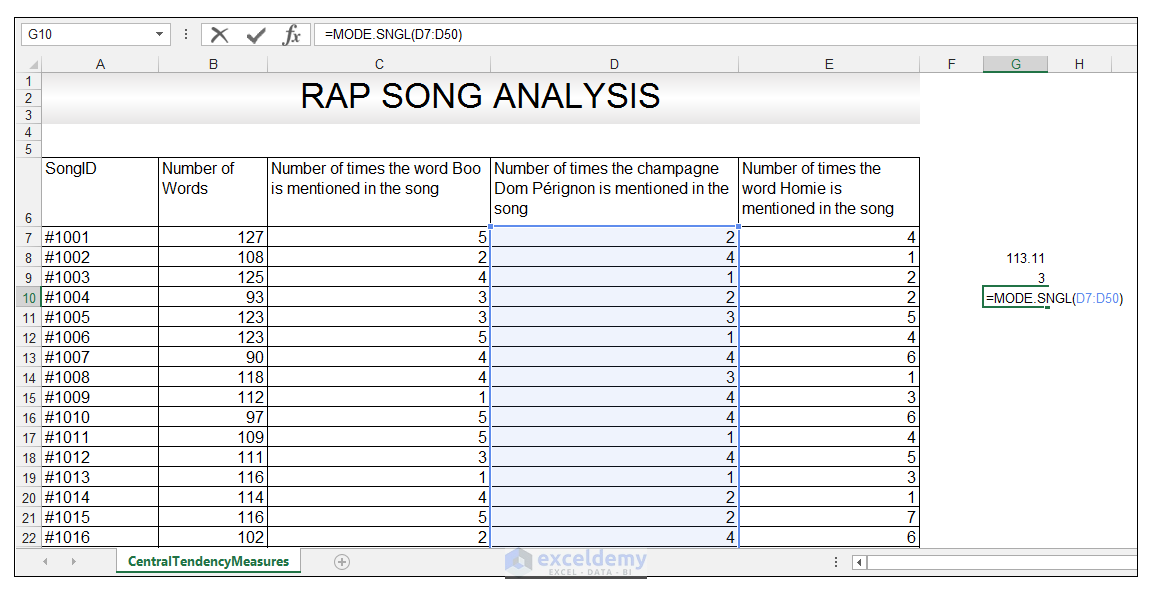The mode function delivers the value of 4, which means that the most often repeated value in the Number of times the champagne Dom Pérignon is mentioned in the song column is 4. Songs that mention Dom Pérignon four times, is the mode in other words.

We are now going to look at getting a vertical array, of the most frequently occurring values in the data set using the MODE.MULT function. This function returns more than one result if there are multiple modes in a data set. We are going to use the Number of times the word Homie is mentioned in the song column. We want to see what the MODE.MULT function returns. When using this function one must include several extra cells, in order to accommodate the delivery of more than one value. One must also enter the formula as an array formula, or else the MODE.SNGL is calculated.

So in order to set up this formula, we select and highlight the cell range I12: I20 as shown below: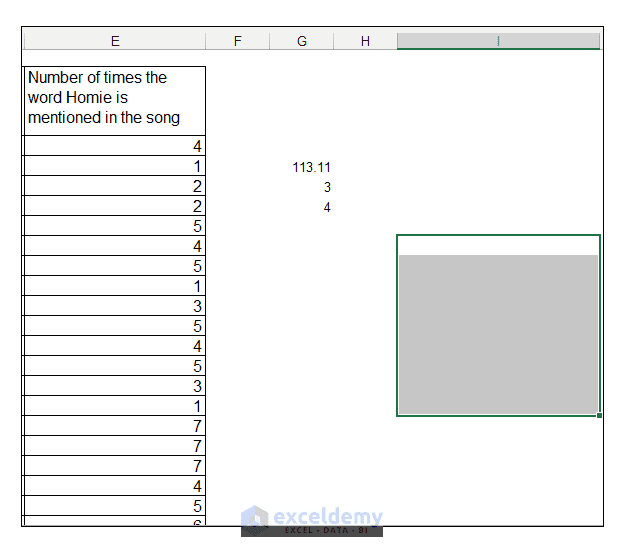Now we type the function in the first cell of the highlighted range in I12:

=MODE.MULT(E7: E50)

and very important because this is an array formula we now press CTRL-SHIFT-ENTER.

We get three modes returned for this dataset, using the MODE.MULT function as shown below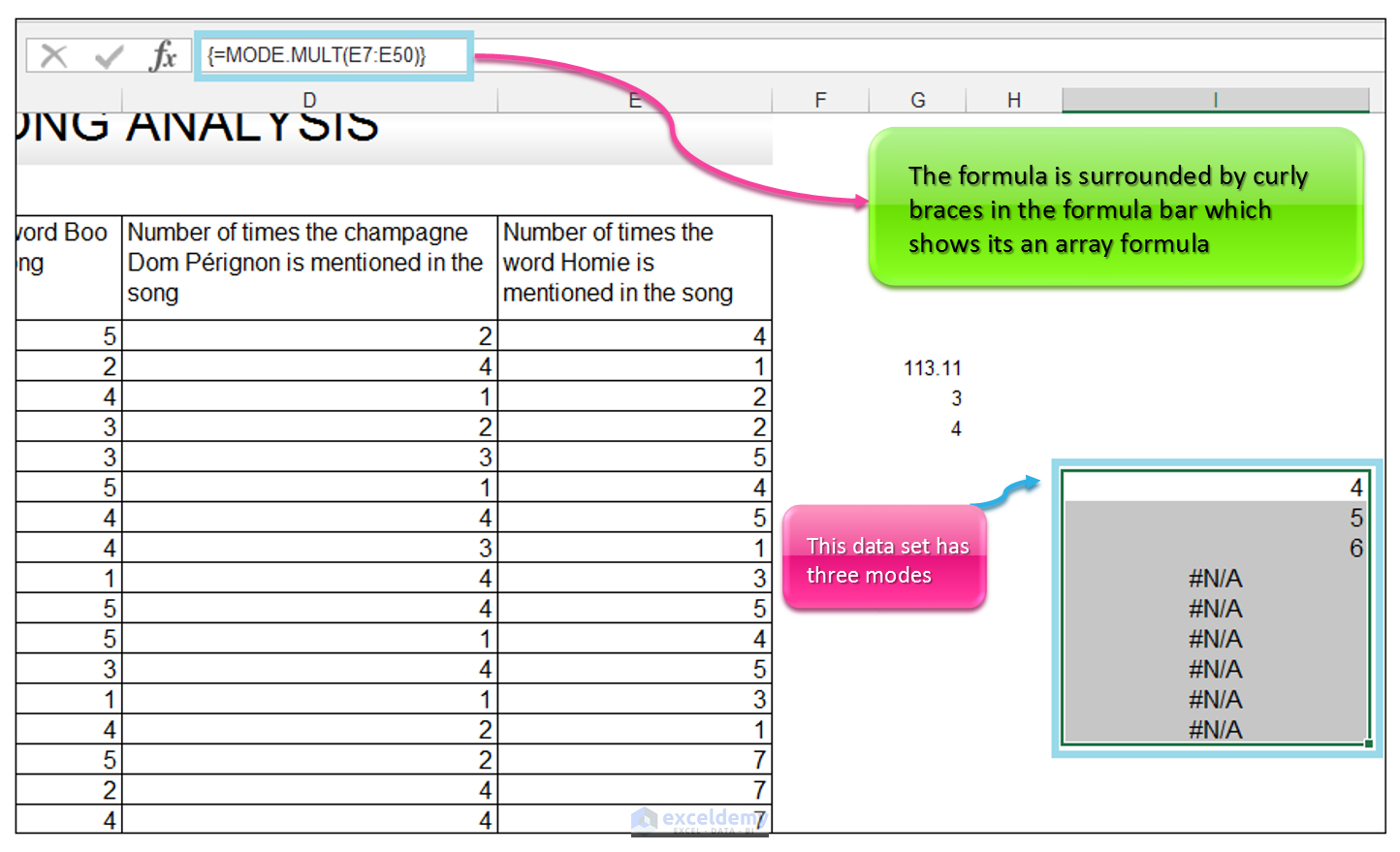We have three modes for this data set, which is 4, 5, and 6.

We can prove this to ourselves, by quickly setting up a COUNTIF to tell us the number of times values appear.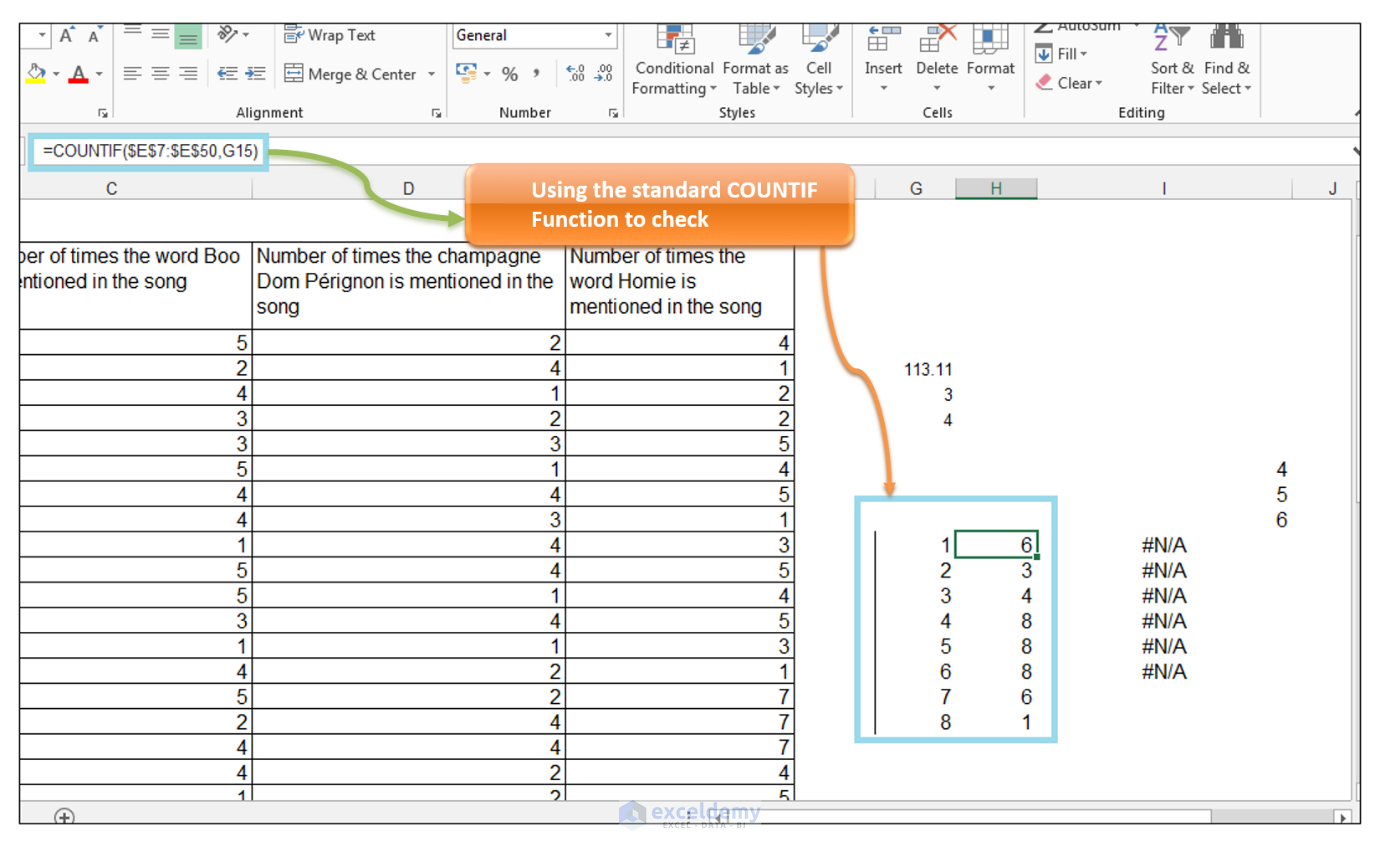When using the COUNTIF to count the number of times each number appears in the data set, we get that 4,5 and 6 each appeared eight times in the data set respectively and thus they are the three modes.

The music producer can thus direct his songwriting team on the best practices, with regards to certain statistical indicators, for writing hit rap songs.

Average-Median-Mode-Mode-Mult.xlsx

## Conclusion

The average, median and mode can give one an overview of data. For a symmetrical distribution of a group of numbers, the mean, median and mode are all the same. For skewed distributions, they differ for the most part. Excel has further enhanced the mode capabilities, due to the introduction of the MODE.MULT function which accommodates more than one mode for a data set.

Please feel free to comment on your views about Hip Hop as a cultural phenomenon and any Excel related analysis you have done on Hip Hop.